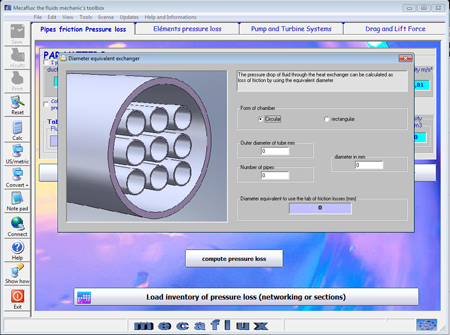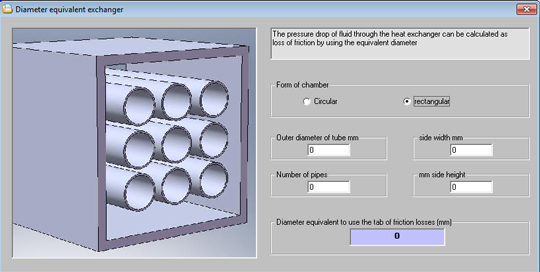Pressure drop and head loss calculation in an exchanger• Calculating the pressure drop across a heat exchanger uses the equivalent diameter:
By entering the dimensions of the heat exchanger (the rectangular or cylindrical shape, width, diameter and the number of internal tubes), you will get an equivalent diameter and you will just have to start the calculation of friction loss in pipes (major head loss) indicating the length of the exchanger and the equivalent diameter.The pressure drop is the calculated pressure drop experienced by the fluid passing through the heat exchanger around the tubes..
• If you want to know the pressure drop of the fluid through the tubes, it suffices to calculate the pressure drop in one tube (onglet pertes de charge reguliere).
Pour rappel:
• If the tubes are in parallel load losses are balanced by distributing the flow rate, the pressure drop of all tubes is equal to the pressure drop of 1 tube.
• the head loss are added only if the tubes are in series (one after another)